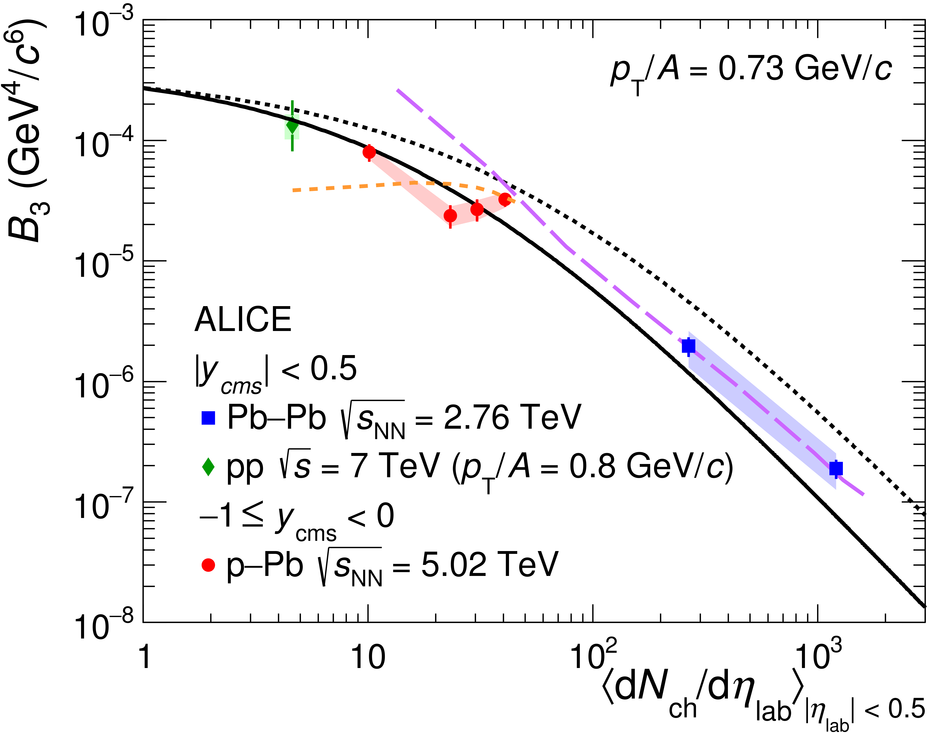# Figure 11

 The coalescence parameter $B_3$, calculated using the average of $^3$He and $^3\overline{\mathrm{He}}$, is shown as a function of the mean charged-particle multiplicity density for $\pt/A = 0.73$ GeV/$c$ (left) and $\pt/A = 0.90$ GeV/$c$ (right) The coalescence parameter is shown with its statistical (line) and systematical (shaded area) uncertainties. In addition, the expectations from the coalescence and the SHM plus Blast-Wave approaches are shown .